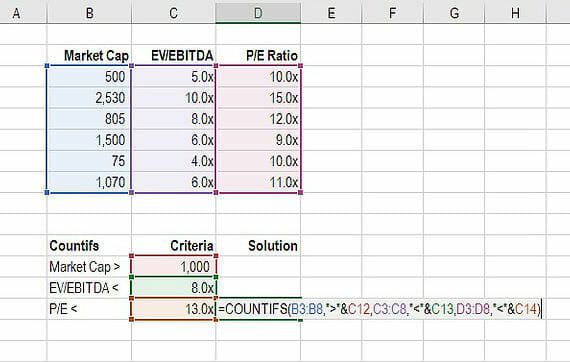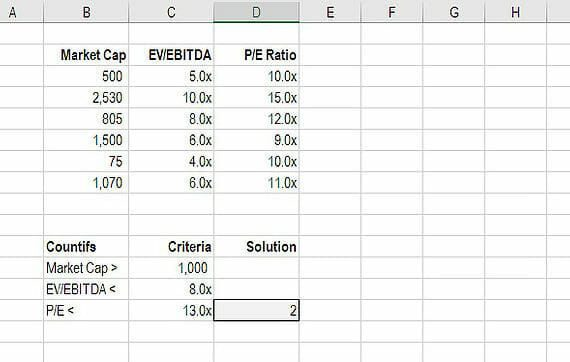# Countif Multiple Criteria

Countifs function in Excel to count cells where multiple criteria are met

## How to countif multiple criteria?

The Excel formula to countif multiple criteria is =countifs(). The “s” on the end makes it plural and therefore implies that there are multiple criteria (2 or more).

In the examples and illustrations below in this tutorial will show you exactly how to use COUNTIFS in Excel.

### How to use countif multiple criteria

Countifs works just like countif, except you can add additional criteria separated by commas. Here is step-by-step instruction on how to do it.

Step 1: document the criteria or conditions you wish to test for
Step 2: type “=countifs(“ and select the range you want to test the first criteria on
Step 3: input the test for the criteria
Step 4: select the second range you want to test (it can be the same range again, or a new one)
Step 5: input the test for the criteria
Step 6: repeat above as many times as necessary
Step 7: close “)” and press enter

### Example of countif with multiple criteria

This screenshot shows counting with multiple conditions in action. As you will see, each of the criteria are being tested and Excel is counting the number of times the result from the test is true.  This is how to use Excel countif multiple criteria:### Why use countifs?

There are many instances where you might want to count the number of times multiple criteria are met. In finance, this can be used to select companies that fit a certain profile. As in the case above, we wanted to find out how many companies were over a certain Market Cap, but below a certain EV/EBITDA multiple and below a certain PE ratio.

### More Excel formulas and functions

Thank you for reading the CFI guide to countif multiple criteria in Excel. If you want to become an Excel power user, we’ve generated a large Excel resources database of all the most important formulas and functions, Excel shortcuts, and general best practices.

Some of our most useful spreadsheet resources include:

• Free Excel crash course
• Excel templates